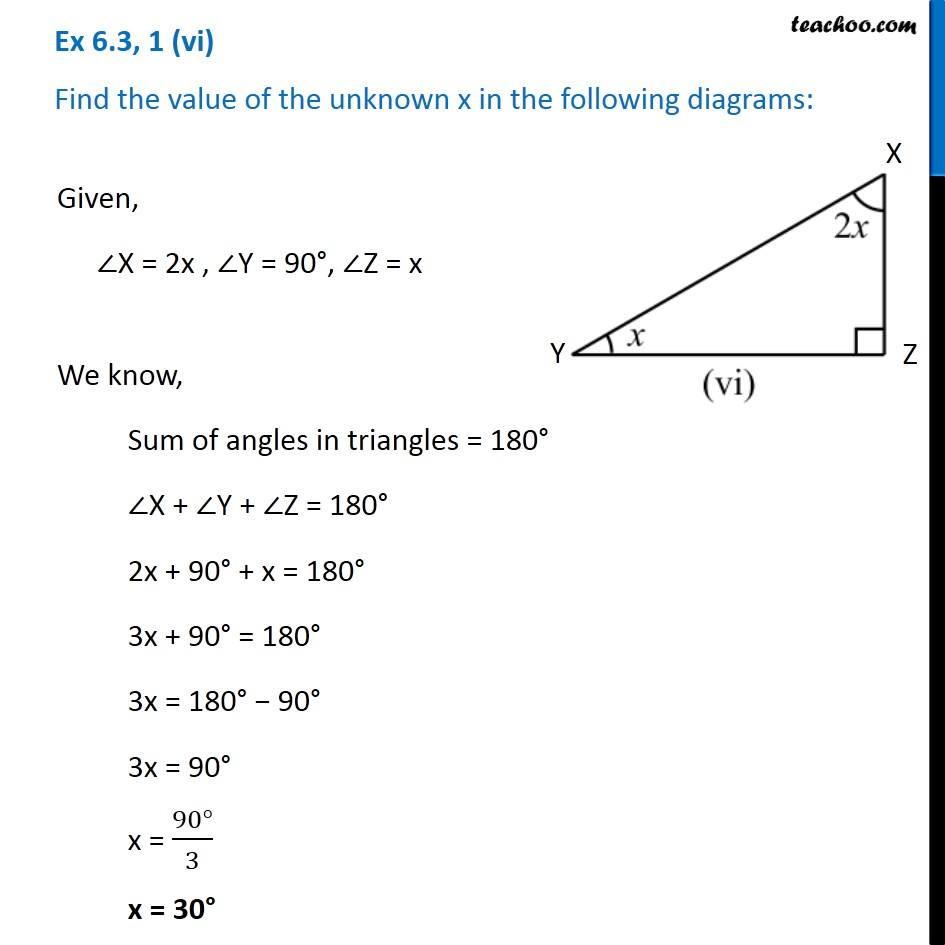Ex 6.3

Chapter 6 Class 7 Triangle and its Properties
Serial order wiseGet live Maths 1-on-1 Classs - Class 6 to 12

### Transcript

Ex 6.3, 1 (vi) Find the value of the unknown x in the following diagrams: Given, ∠X = 2x , ∠Y = 90°, ∠Z = x We know, Sum of angles in triangles = 180° ∠X + ∠Y + ∠Z = 180° 2x + 90° + x = 180° 3x + 90° = 180° 3x = 180° − 90° 3x = 90° x = (90°)/3 x = 30°